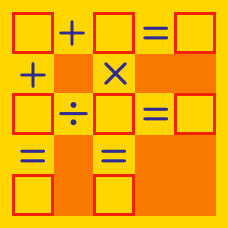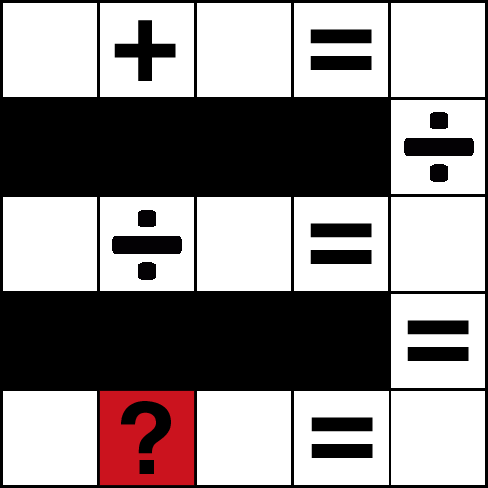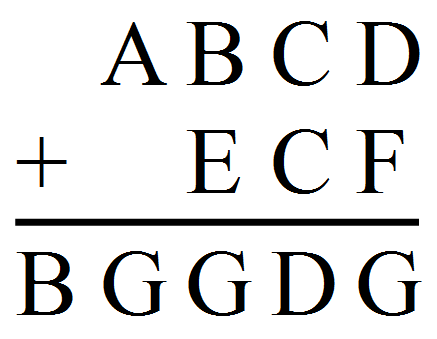Logic

# Logical Arithmetic Warmup

$\square6 + \square7 = 1\square3$ All three boxes contain the same digit. What digit belongs in the boxes to make this equation true?

This is an arithmetic puzzle, where $1 \square$ would represent the 2-digit number 19 if $\square = 9$. It does not represent the algebraic expression $1 \times \square$.

I am thinking of a three digit number. The product of the digits in my number is 14, and the last digit is twice as big as the first digit.

What number am I thinking of?

A certain five-digit number has the property that if the digit 1 is placed (concatenated) on the right side of the number, the result is three times larger than the value that results if the digit 1 is placed at the beginning of the number.

What number is it?The red square in the above grid should contain an operator $(+, -, \times, \div)$ and the white squares should contain the numbers 1 through 9 such that each number appears once and reading across each row and down the righthand column make true statements.

What operation goes into the red square?In the above image, each letter represents a unique digit.

What digit does the letter F represent?

×